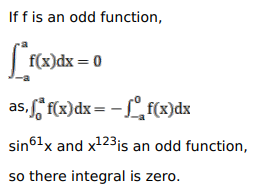# Mark against the correct answer in the following:Question:

Mark $(\sqrt{)}$ against the correct answer in the following:

$\int_{-\pi}^{\pi}\left(\sin ^{61} x+x^{123}\right) d x=?$

A. $2 \pi$

B. 0

C. $\frac{\pi}{2}$

D. $125 \pi$

Solution: